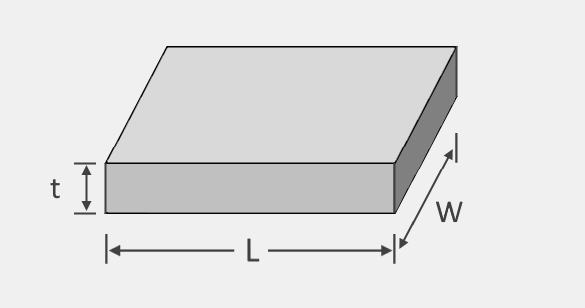# Plate Weight Calculator

Created by Kenneth Alambra
Reviewed by Dominik Czernia, PhD and Jack Bowater
Last updated: Jan 20, 2023

This plate weight calculator is our version of the metal weight calculator for metals in a plate shape. Don't worry, it covers plates of other materials too! This calculator will help you determine the weight of any plate, no matter its area, thickness, or density.

In this calculator, you will learn how to calculate the plate weight of any material, be a metal plate weight, a ceramic plate weight, a steel plate weight, or any others. Keep on reading to learn more.

## What is a plate in construction?

A plate is a material shape obtained either by rolling, hammering, or forging malleable materials, such as most metals and some plastics, or by casting brittle materials, like ceramics, glass, and other brittle plastics and metals.

In construction, we use plates in the fabrication of other products by combining them with other materials. We can cut and forge metal plates and weld or join them together to form other complex products. On the other hand, we use brittle plate materials for other miscellaneous uses, such as insulation materials, decoration, and other special purposes.

In electronics, we can layer multiple plates of various metals and submerge them in a liquid electrolyte to make a wet cell battery. We also use capacitive plates separated by a dielectric plate to make parallel plate capacitors.

Most of the time, a plate is made with a uniform thickness throughout the material. This characteristic then results in uniform properties of the material and is also a good reference when determining its weight.

## Plate weight calculator: How to calculate plate weight?

We need to determine or estimate a plate's weight to know if the material suits our needs. It would be helpful to know the weight for logistics purposes and structural analysis, especially larger size plates.

To calculate plate weight, we have to multiply the surface area of our plate by its thickness to obtain its volume. After this, we need to multiply this volume by the plate material's density to get its weight. We can write this as an equation:

plate weight = plate area × plate thickness × density of material

You can use our area of a rectangle calculator or generic area calculator and our density calculator to find the necessary data for every situation that requires you to calculate the weight of a plate.

Let us try to use this equation on a sample calculation to find out how much a particular plate weighs in this text's next section.

## Sample calculation of plate weight

Let's say we need to calculate the weight of two rectangular pieces of 0.8 m x 2.4 m x 5.0 mm (or 0.005 m) thick lead plates** for the fabrication of a [radiation-proof door. Considering the lead density of 11,340 kg/m³, we can then calculate the weight of a single plate as follows:

plate weight = plate area × plate thickness × density of material

plate weight = 0.8 m × 2.4 m × 0.005 m × 11,340 kg/m³

plate weight = 0.0096 m³ × 11,340 kg/m³

plate weight = 108.864 kg

From our calculation above, we now know that one piece of the lead plate needed for the radiation-proof door weighs **108.864 kg**. Since we need two of these plates, we have to multiply this value by 2 to obtain the total weight of 108.864 kg × 2 plates = **217.728 kg**`.

If you want to know how to calculate plate weight for other materials, use our plate weight calculator where we have gathered the densities for plenty of metals.

If in case you needed to determine the weight of materials made of specific steel alloys, check our steel weight calculator to also learn more about steel. You can also check our aluminum weight calculator if you want to learn about a much lighter metal like aluminum.

Kenneth Alambra
Material
Silver
Density of material
lb/cu ft
Shape of the plate
RectangularPlate details
Length (L)
in
Width (W)
in
Area
in²
Thickness (t)
in
Volume
cu in
Quantity of plates
Final output
Total weight
lb
People also viewed…

### BMR - Harris-Benedict equation

Harris-Benedict calculator uses one of the three most popular BMR formulas. Knowing your BMR (basal metabolic weight) may help you make important decisions about your diet and lifestyle.

### Flat vs. round Earth

Omni's not-flat Earth calculator helps you perform three experiments that prove the world is round.

### Grout

This grout calculator will help you determine the amount of tile grout needed for your next tiling project.